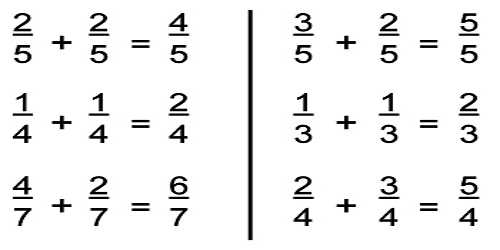MathematicA fraction represents a part of a whole or, more generally, any number of equal parts. A common denominator must be found when adding fractions that have different denominators. This is the most important (and probably the hardest) step in adding or subtracting fractions. A common denominator can always be found by multiplying the denominators.

Adding Fractions with the Same Denominator

Fractions consist of two numbers. The top number is called the numerator. The bottom number is called the denominator.

numerator / denominator

To add two fractions with the same denominator, add the numerators and place that sum over the common denominator.

How to Add Fractions with different denominators:

• Find the Least Common Denominator (LCD) of the fractions
• Rename the fractions to have the LCD
• Add the numerators of the fractions
• Simplify the Fraction

Example: Find the Sum of 2/9 and 3/12

• Determine the Greatest Common Factor of 9 and 12 which is 3
• Either multiply the denominators and divide by the GCF (9*12=108, 108/3=36)
• OR – Divide one of the denominators by the GCF and multiply the answer by the other denominator (9/3=3, 3*12=36)
• Rename the fractions to use the Least Common Denominator(2/9=8/36, 3/12=9/36)
• The result is 8/36 + 9/36
• Add the numerators and put the sum over the LCD = 17/36
• Simplify the fraction if possible. In this case, it is not possible

Adding Mixed Numbers with the Same Denominator

Mixed numbers consist of an integer followed by a fraction.

• How to add two mixed numbers whose fractions have the same denominator:
• Add the numerators of the two fractions
• Place that sum over the common denominator.
• If this fraction is improper (numerator larger than or equal to the denominator) then convert it to a mixed number
• Add the integer portions of the two mixed numbers
• If adding the fractional parts created a mixed number then add its integer portion to the sum.

Information Source: